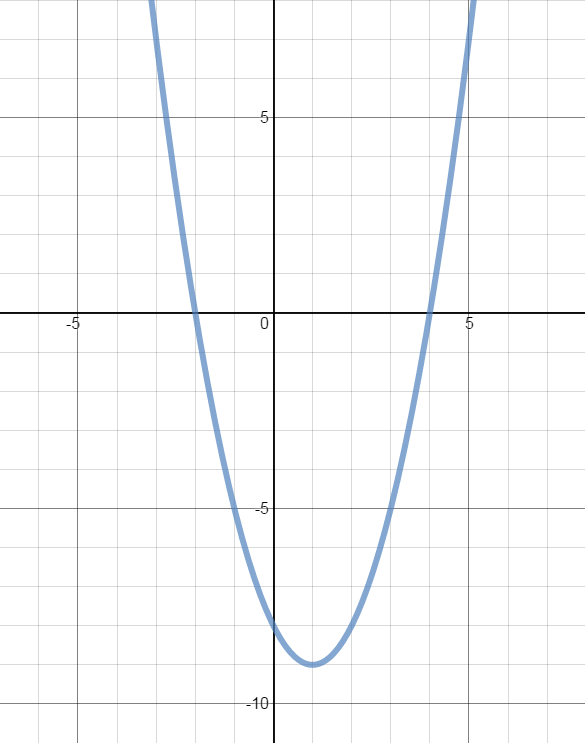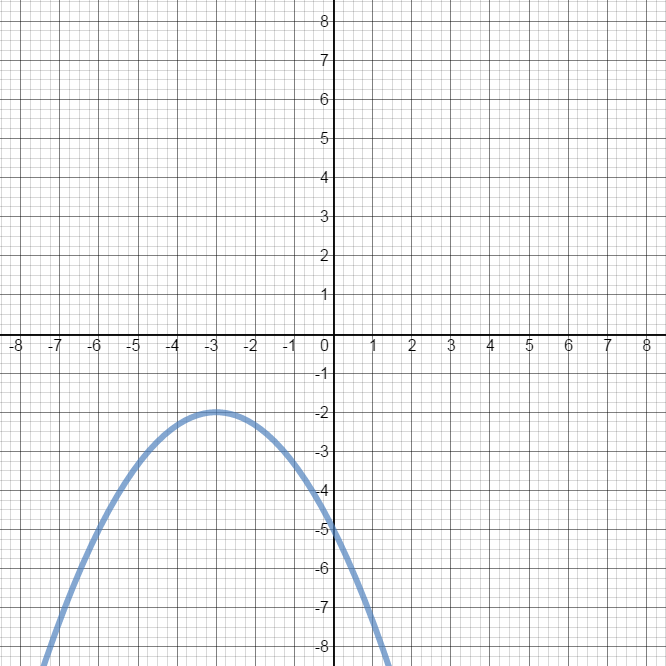0/10
##### Examples
###### Lessons
1. Determining the Characteristics of a Quadratic Function Using Various Methods

Determine the following characteristics of the quadratic function $y = -2x^2 + 4x + 6$:

• Opening of the graph

$y-$intercept

$x-$intercept(s)

• Vertex

• Axis of symmetry

• Domain

• Range

• Minimum/Maximum value

1. Using factoring
3. Using completing the square
4. Using the vertex formula
2. From the graph of the parabola, determine the:
• vertex
• axis of symmetry
• y-intercept
• x-intercepts
• domain
• range
• minimum/maximum value

1.2.3. Identifying Characteristics of Quadratic function in General Form: $y = ax^2 + bx+c$
$y = 2{x^2} - 12x + 10$ is a quadratic function in general form.

i) Determine:
• y-intercept
• x-intercepts
• vertex

ii) Sketch the graph.
1. Identifying Characteristics of Quadratic Functions in Vertex Form: $y = a(x-p)^2 + q$
$y = 2{\left( {x - 3} \right)^2} - 8$ is a quadratic function in vertex form.

i) Determine:
• y-intercept
• x-intercepts
• vertex

ii) Sketch the graph.
###### Free to Join!
StudyPug is a learning help platform covering math and science from grade 4 all the way to second year university. Our video tutorials, unlimited practice problems, and step-by-step explanations provide you or your child with all the help you need to master concepts. On top of that, it's fun - with achievements, customizable avatars, and awards to keep you motivated.
• #### Easily See Your ProgressWe track the progress you've made on a topic so you know what you've done. From the course view you can easily see what topics have what and the progress you've made on them. Fill the rings to completely master that section or mouse over the icon to see more details.
• #### Make Use of Our Learning Aids###### Practice Accuracy

See how well your practice sessions are going over time.

Stay on track with our daily recommendations.

• #### Earn Achievements as You LearnMake the most of your time as you use StudyPug to help you achieve your goals. Earn fun little badges the more you watch, practice, and use our service.
• #### Create and Customize Your AvatarPlay with our fun little avatar builder to create and customize your own avatar on StudyPug. Choose your face, eye colour, hair colour and style, and background. Unlock more options the more you use StudyPug.
###### Topic Notes
Three properties that are universal to all quadratic functions: 1) The graph of a quadratic function is always a parabola that either opens upward or downward (end behavior); 2) The domain of a quadratic function is all real numbers; and 3) The vertex is the lowest point when the parabola opens upwards; while the vertex is the highest point when the parabola opens downward.